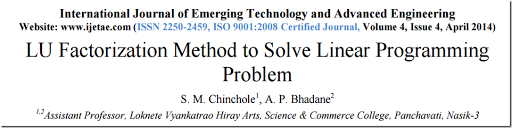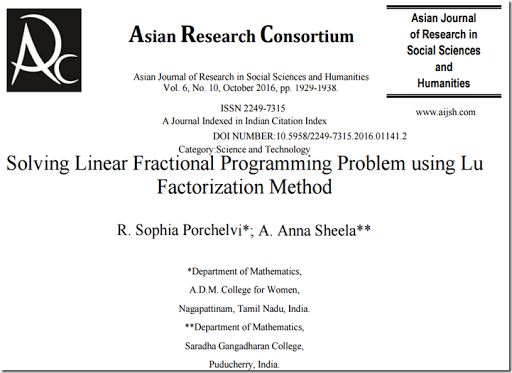## Tuesday, January 3, 2017

### Solving LP by LU???

Yes, according to this paper [link]:Abstract This paper presents a new approach for the solution of Linear Programming Problems with the help of LU Factorization Method of matrices. This method is based on the fact that a square matrix can be factorized into the product of unit lower triangular matrix and upper triangular matrix. In this method, we get direct solution without iteration. We also show that this method is better than simplex method.

Basically the paper claims that we can solve an LP

 \begin{align}\max\>& c^Tx\\&Ax \le b \\& x\ge 0\end{align}

as follows:

1. Make sure $$A$$ is square (by adding rows or column if needed using vacuous constraints or extra slacks).
2. Solve $$Ax=b$$ using LU decomposition
3. Done

Wow. This is baloney of course.  But here is someone actually believing this stuff.

##### Update

Of course we can use this nonsensical method and apply it to a different problem:Abstract. In this paper the linear fractional programming problem is converted to a linear programming problem and for solving it, LU Factorization method is introduced. Finally, to illustrate the proposed method, it is verified by means of the numerical example and the results conclude that this method gives more reliable solution or the equal solution compared to the existing method.

My goodness.

#### References

1. S.M.Chincole, A.P.Bhadane, LU Factorization Method To Solve Linear Programming Problem, International Journal of Emerging Technology and Advanced Engineering ,Volume 4, Issue 4, 176-180, http://www.ijetae.com/files/Volume4Issue4/IJETAE_0414_31.pdf. Warning: this is nonsensical. Note that the example has all constraints binding in the optimal solution.
2. R. Sophia Porchelvi, A. Anna Sheela, Solving Linear Fractional Programming Problem Using LU Factorization Method, Asian Journal of Research in Social Sciences and Humanities Vol. 6, No. 10, October 2016, pp. 1929-1938, https://www.aijsh.com/shop/articlepdf/2016/10/1475416103154.pdf. Warning squared: astonishingly this is a paper based on (1).